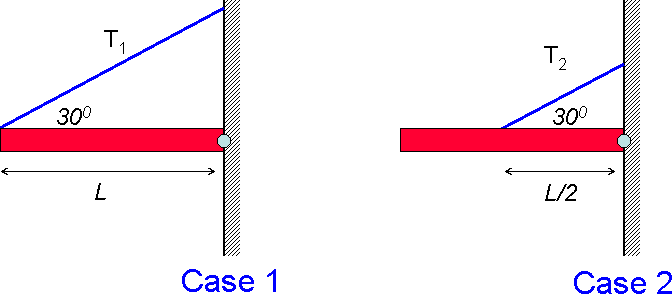# Problem: In both cases the beam has the same mass and length and is attached to the wall by a hinge.1) In which of the static cases shown is the tension in the supporting wire bigger?A) Case 1B) Case 2C) Same

###### FREE Expert Solution

Torque:

Rotational equilibrium:

$\overline{){\mathbf{\Sigma }}{\mathbf{\tau }}{\mathbf{=}}{\mathbf{0}}}$

Take counterclockwise as positive.

Case 1:

mg(L/2) - T1•L•sin 30 = 0

87% (462 ratings)###### Problem DetailsIn both cases the beam has the same mass and length and is attached to the wall by a hinge.

1) In which of the static cases shown is the tension in the supporting wire bigger?

A) Case 1

B) Case 2

C) Same